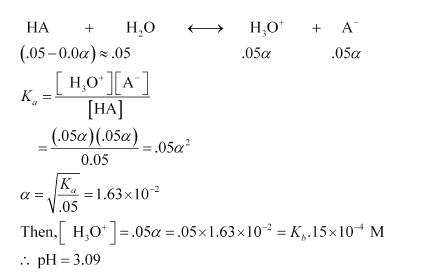# The ionization constant of propanoic acid is 1.32 × 10–5.

Question:

The ionization constant of propanoic acid is $1.32 \times 10^{-5}$. Calculate the degree of ionization of the acid in its $0.05 \mathrm{M}$ solution and also its pH. What will be its degree of ionization if the solution is $0.01 \mathrm{M}$ in $\mathrm{HCl}$ also?

Solution:

Let the degree of ionization of propanoic acid be α.

Then, representing propionic acid as HA, we have:In the presence of 0.1M of HCl, let α´ be the degree of ionization.

Then, $\left[\mathrm{H}_{3} \mathrm{O}^{+}\right]=0.01$

$\left[\mathrm{A}^{-}\right]=005 \alpha^{\prime}$

$[\mathrm{HA}]=.05$

$K_{a}=\frac{0.01 \times .05 \alpha^{\prime}}{.05}$

$1.32 \times 10^{-5}=.01 \times \alpha^{\prime}$

$\alpha^{\prime}=1.32 \times 10^{-3}$